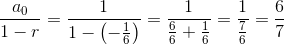# High School Math : Finding Sums of Infinite Series

## Example Questions

### Example Question #1 : Sums Of Infinite Series

Find the value for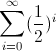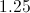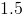Explanation:

To best understand, let's write out the series. So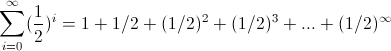We can see this is an infinite geometric series with each successive term being multiplied by.

A definition you may wish to remember is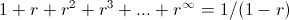where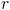stands for the common ratio between the numbers, which in this case isor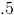. So we get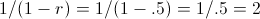### Example Question #2 : Sums Of Infinite Series

Evaluate: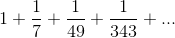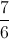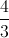The series does not converge.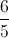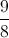Explanation:

This is a geometric series whose first term is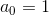and whose common ratio is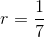. The sum of this series is: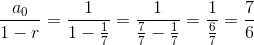### Example Question #3 : Sums Of Infinite Series

Evaluate: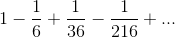The series does not converge.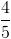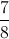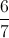This is a geometric series whose first term isand whose common ratio is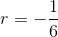. The sum of this series is: